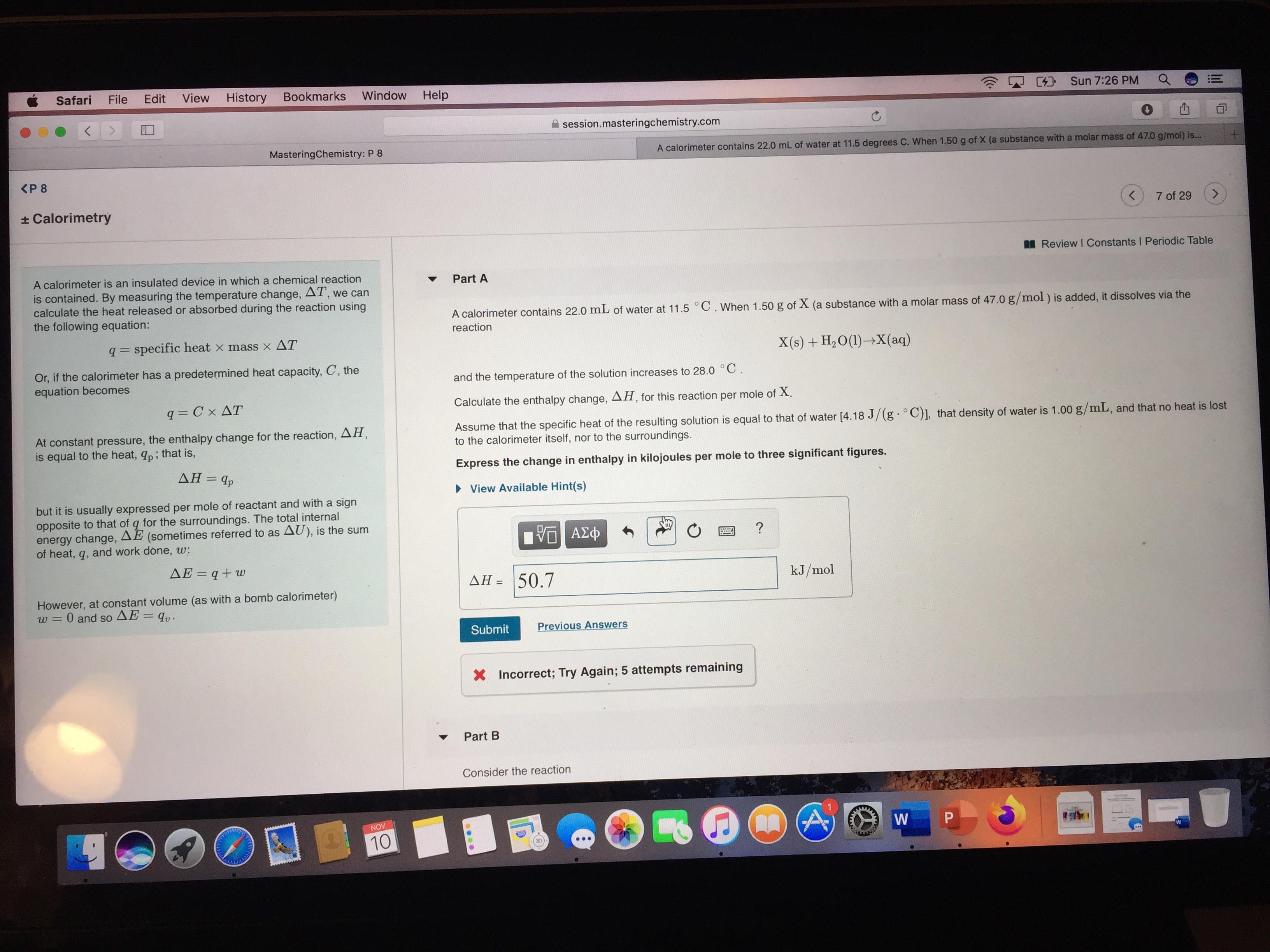# A calorimeter contains 22.0 mL of water at 11.5°C. When 1.50 g of X (a substance with a molar mass of 47.0 g/mol) is added, it dissolves via the reaction X(s) + H2O → X(aq) and the temperature of the solution increases to 28.0°C. Calculate the enthalpy change ∆G for this reaction per mole of X. Assume that the specific heat of the solution is equal to that of water (4.18J/g•°C), that the density oof water is 1.00 g/mL, and that no heat is lost to the calorimeter itself, nor to the surroundings